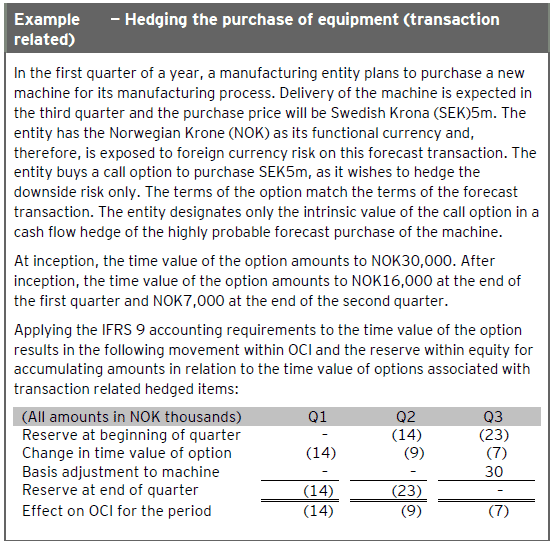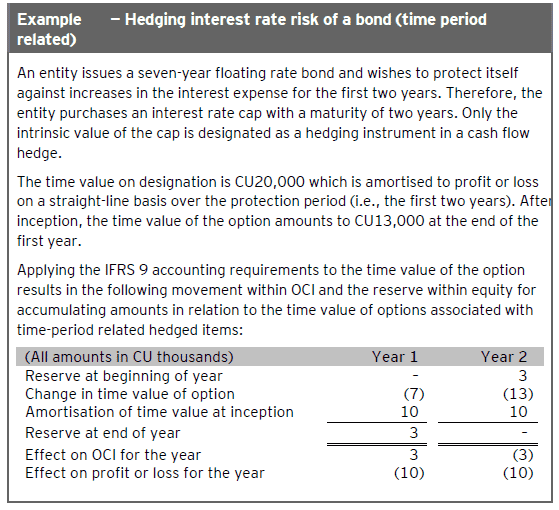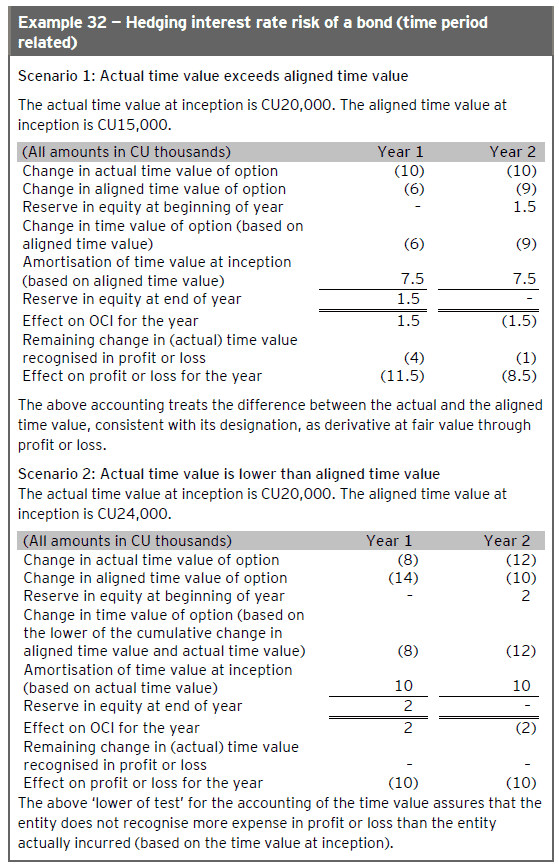# Time value of options

## Other changes from IAS 39

The fair value of an option consists of the intrinsic value and the time value. When using an option for hedging activities, only the intrinsic value is used for offsetting the fair value changes attributable to the hedged risk (unless the hedged item is also an option, see ‘Hedged items‘). Unchanged from IAS 39, an entity can either designate an option as a hedging instrument in its entirety, or it can separate the intrinsic value and the time value and designate only the

Under IAS 39, when designating the option in its entirety as a hedge of a non-option item, changes in the portion of the fair value attributable to the time value result in ineffectiveness. Depending on the level of ineffectiveness, an entity might even not pass the prospective effectiveness assessment or be forced to discontinue hedge accounting as a result of changes in the time value. Alternatively, when designating the intrinsic value of the option only, the time value has to be accounted for at fair value through profit or loss, thus, also resulting in potentially significant profit or loss volatility. In either case, the change in the time value will be recognised in profit or loss.

From a risk management perspective, entities typically consider the premium paid on an option (which, on inception, is often only time value) as a cost of hedging rather than a trading position. Economically, the time value could be considered as a premium for protection against risk (i.e., an ‘insurance premium’).

IFRS 9 does not change how an option is designated in a hedging relationship (i.e., whether in its entirety or the intrinsic value only). However, the IASB has acknowledged these concerns and introduced a new accounting treatment for changes in the fair value of the time value if only the intrinsic value is designated in the hedging relationship.

Changes in the fair value of the time value of options are first recognised in OCI. The subsequent treatment depends on the nature of the hedged transaction.

The standard differentiates between transaction related hedged items and time-period related hedged items:

• Transaction related hedged item: the time value of an option used to hedge such an item represents part of the cost of the transaction. An example would be a hedge of a forecast commodity purchase. The amount that is accumulated in OCI is removed similar to amounts accumulated in the cash flow hedge reserve (see ‘Cash flow hedges‘), i.e., if the hedged transaction subsequently results in the recognition of a non-financial item the amount becomes a ‘basis adjustment’ and else the amount is reclassified to profit or loss (reclassification adjustment) in the same period or periods during which the hedged cash flows affect profit or loss.
• Time-period related hedged item: the time value of an option used to hedge such an item has the character of the cost of protection against a risk over a particular period of time. An example would be a hedge of commodity inventory over a six month period. The amount that is accumulated in OCI is amortised on a systematic and rational basis to profit or loss as a reclassification adjustment. The amortisation period is the period during which the hedge adjustment for the option’s intrinsic value could affect profit or loss (or other comprehensive income in case the option hedges an equity instrument accounted for at fair value through other comprehensive income).

The distinction between transaction related hedged items and time-period related hedged items reflects that the accounting for the time value of the option should follow general IFRS principles for how to account for payments that are akin to insurance premiums (the ‘insurance premium view’ mentioned above). So, in making the distinction, an entity needs to consider how the accounting for the hedged item will eventually affect profit or loss.

If the hedged item later results in a transaction for which the transactions costs are accounted for as part of a one-off event (like a purchase or a sale of an item), the option’s time value relates to a transaction related hedged item.

Examples are hedges of forecast purchases of inventory or property, plant and equipment, and forecast sales. Similarly, if the purchases or sales result from a firm commitment, the option’s time value relates to a transaction related hedged item because the transactions resulting from those firm commitments affect profit or loss at the same time as similar forecast transactions.

If the hedged item later results in protection against risk for a particular period that does not involve a transaction for which the transactions costs are accounted for as part of a one-off event, the option’s time value relates to a time-period related hedged item. Examples are hedges of interest expense or income in particular periods, already existing inventory hedged for fair value changes or a hedge of a net investment in a foreign operation. In the case of a forward starting interest rate option, the time value would be amortised over the interest periods that the option covers (i.e. the amortisation period would exclude the initial part of the option’s life).

A practical reference point for identifying the correct accounting is to look at how the intrinsic value of the related hedging relationship could affect profit or loss. That dictates the timing of recognising the time value of the option in profit or loss in most cases, with firm commitments as hedged items being the notable exception. For fair value hedges of firm commitments to buy or sell a non-financial item, any intrinsic value of the option would affect profit or loss during the commitment period but the time value of the option would still have to be deferred until the commitment is settled (as noted above for transaction-related hedged items).

It is important to note that because this accounting for ‘costs of hedging’ only applies if the time value of the option is excluded from the designation of the hedging relationship, the amounts deferred in accumulated other comprehensive income are not part of the cash flow hedge reserve but instead a different component of equity. The cash flow hedge reserve only includes amounts that are gains or losses on hedging instruments that are determined to be an effective hedge (i.e., amounts that are included in the designation of a hedging relationship). By default, the time value will be zero at the expiry of an option contract. For a transaction related hedged item, recognising the fair value changes of the time value in OCI means that on expiry, the time value that existed at designation will have accumulated in OCI. Once the hedged transaction happens, the accounting for the accumulated time value follows the accounting for any changes in fair value of the intrinsic value of the option (that were also accumulated in OCI). For time-period related hedged items, the standard does not prescribe what ‘on a systematic and rational basis’ means in the context of amortising the time value from OCI to profit or loss. A straight-line amortisation is appropriate in most cases.The accounting for the time value of options would also apply to combinations of options, for example, when hedging a highly probable forecast transaction with a zero-cost collar. When designating the intrinsic value only, the volatility resulting from changes in the time values of the two options would be recognised in other comprehensive income. However, the amortisation (in the case of time-period related hedged items) or the transaction costs deferred at the end of the life of the hedging relationship (for transaction-related hedged items) would be nil when using a zero-cost collar.

The two examples above both assume that the critical terms of the option match the hedged item. However, in practice, this is not always the case. The accounting treatment described above applies only to the extent the time value relates to the hedged item. An additional assessment has to be made if the critical terms of the option do not match the hedged item. For that purpose, the actual time value has to be compared with that of a hypothetical option that perfectly matches the critical terms of the hedged item (in IFRS 9 referred to as the aligned time value).

When the terms of the option are not aligned with the hedged item, the accounting for the time value in situations in which the aligned time value exceeds the actual time value is different to situations in which the actual time value exceeds the aligned time value.

If, at inception, the actual time value exceeds the aligned time value:

• The aligned time value at inception is amortised on a rational basis from OCI to profit or loss over the period the hedged item affects profit or loss (for a time-period related hedged item).
• The change in the fair value of the aligned time value is recognised in OCI.
• The remaining difference in change in fair value between the actual time value and the aligned time value is recognised in profit or loss.

If, at inception, the aligned time value exceeds the actual time value:

• The actual time value at inception is amortised on a rational basis from OCI to profit or loss over the period the hedged item affects profit or loss (for a time-period related hedged item).
• Lower of the cumulative change in the fair value of the actual time value and the aligned time value is recognised in OCI.
• The remaining difference in change in fair value between the actual time value and the aligned time value, if any, is recognised in profit or loss.

For the hedging strategy introduced in the second example above, this would change the accounting, as follows:IFRS 9 does not define the ‘aligned time value’ in much detail but it is clear that it is part of the concept of ‘costs of hedging’. Therefore, regular pricing features, such as dealer margins, are part of the aligned time value of an option, reflecting that they are part of the fair value of the financial instrument whose intrinsic value is designated as the hedging instrument.

This is different from using a hypothetical derivative, which has the purpose of measuring the hedged item. For that purpose, features that are only in the hedging instrument but not the hedged item cannot be taken into account, whereas the same rationale does not apply for the purpose of accounting for the costs of hedging. This becomes clearer from the example of the foreign currency basis spread (see ‘Foreign currency basis spreads‘); it cannot be included as part of a hypothetical derivative to measure the hedged item but it is a cost of hedging.